# Evaluate and Use Negative Indices

In this worksheet, students will evaluate and calculate negative indices with negative numbers and fractions.Key stage:  KS 4

GCSE Subjects:   Maths

GCSE Boards:   Pearson Edexcel, OCR, Eduqas, AQA

Curriculum topic:   Number, Indices and Surds

Curriculum subtopic:   Structure and Calculation, Powers and Roots

Difficulty level:### QUESTION 1 of 10

If you confident with positive integers, we are now going to learn to evaluate numbers using negative indices.

If you are not sure what these are, why not review these before you move forwards?

Think about the patterns you can see in the following table:

 Start with 1000 1000 10 x 10 x 10 103 Then divide by 10 100 10 x 10 102 Divide by 10 again 10 10 101 Divide by 10 again 1 1 100  Remember that any term to the power of 0 is 1 Divide by 10 again10-1 Divide by 10 again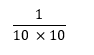10-2 Divide by 10 again10-3

We can see that the pattern in these powers of 10 shows us that:Notice that we are, in fact, finding the 'reciprocal' of:e.g. Evaluate 2-3.

This means work out the value of:We have found the reciprocal of 23.

e.g. Evaluate 3-4.

This means work out the value of:We have found the reciprocal of 34.

In this activity, we will evaluate and calculate negative indices with negative numbers and fractions as we have seen in the examples above.

You may want to have a pen and paper handy before you start, so that you can compare your working to our answers written by a maths teacher.

Evaluate:

2-4

( 2 + -4)

(2 x 2 x 2 x 2)2 x (-4)

Evaluate:

5-3

If you need to use a fraction, write your answer in the form a/b, without spaces and using the / key to create your fractions bar.

e.g. Evaluate (-5)-3.

We need to calculate:This can also be written as:Using this process, can you now evaluate:

(-6)-3

-9

-216

-1 /216

18

We will also need to be able to evaluate powers of fractions.

e.g.can be calculated by finding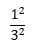So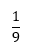Here's another example: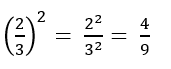Using this information, evaluate:2/25

4/25

4/5

4/7

We can be asked to evaluate negative fractions.

e.g.Remember that when we cube a negative number we should expect a negative answer.Using this knowledge, evaluate:8/27

-8/27

Now we need to look at using negative indices with fractions.

Remember that a negative power involves finding a reciprocal.

This means that: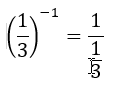To work out 1 divided by a fraction, we need to change the operator to multiplication and invert or 'flip' the fraction, like this:So the correct answer is: 3

Here is another example:If we take this step by step:

1. We know that a negative power means that we need to find the reciprocal of the fraction to the power of 2

2. So we are calculating 1 divided by one-third squared.

3. We follow the rules of dividing fractions and find that 9 is the correct answer.

Using this knowledge, evaluate: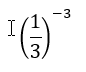Hint: This means we need to work out:So far we have found negative powers of fractions that have a numerator of 1.

We follow the same steps to calculate the negative power of a fraction, that has another number as the numerator.

e.g. Evaluate:This means find the value of: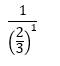As before, we use our fractions skills to work out:Informally, we can see that finding the reciprocal of the fractions has inverted the original fraction (or turned it upside down!)We can use this 'shortcut' when we need to work out fractions with other negative powers.

e.g. Evaluate:=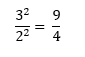Use this process to evaluate:Hint: Write this to start: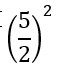Which of the options are equivalent to the fraction index below?Which of the options are equivalent to the fraction index below?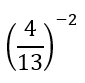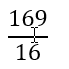Imagine that your friend has evaluated the fraction index below:They have written their answer as:Did they get it right?

Correct

Incorrect

• Question 1

Evaluate:

2-4EDDIE SAYS
In this question, we have been asked to work out 2 to the power of negative 4. This is the same as finding the reciprocal or 1 / (2 to the power of 4). 24 = 16 The reciprocal of 16 is 1/16.
• Question 2

Evaluate:

5-3

If you need to use a fraction, write your answer in the form a/b, without spaces and using the / key to create your fractions bar.

1/125
EDDIE SAYS
We have been asked to work out 53. This is the same as the reciprocal or 1/ 53. 53 = 5 x 5 x 5 = 125 This means the correct answer is:• Question 3

e.g. Evaluate (-5)-3.

We need to calculate:This can also be written as:Using this process, can you now evaluate:

(-6)-3

-1 /216
EDDIE SAYS
We should calculate the reciprocal of -63. -63 = (-6) x (-6) x (-6) If we tackle the first two numbers initially: (-6) x (-6) = 36 Remember that multiplying two negative numbers gives a positive outcome. Then let's move on to the final pair: 36 x (-6) = -216 Remember that multiplying a negative and positive number together gives a negative outcome. The reciprocal of this number is our answer, which is:• Question 4

We will also need to be able to evaluate powers of fractions.

e.g.can be calculated by findingSoHere's another example:Using this information, evaluate:4/25
EDDIE SAYS
We need to square 2 and square 5 and write these answers as a fraction: 2 x 2 = 4 5 x 5 = 25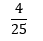• Question 5

We can be asked to evaluate negative fractions.

e.g.Remember that when we cube a negative number we should expect a negative answer.Using this knowledge, evaluate:-8/27
EDDIE SAYS
Remember that cubing a negative number gives a negative answer. Let's work out each element then put them together into a fraction: (-2) x (-2) x (-2) = -8 3 x 3 x 3 = 27 So we know the correct value is: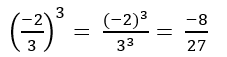• Question 6

Now we need to look at using negative indices with fractions.

Remember that a negative power involves finding a reciprocal.

This means that:To work out 1 divided by a fraction, we need to change the operator to multiplication and invert or 'flip' the fraction, like this:So the correct answer is: 3

Here is another example:If we take this step by step:

1. We know that a negative power means that we need to find the reciprocal of the fraction to the power of 2

2. So we are calculating 1 divided by one-third squared.

3. We follow the rules of dividing fractions and find that 9 is the correct answer.

Using this knowledge, evaluate:Hint: This means we need to work out:27
EDDIE SAYS
We calculate the answer like this: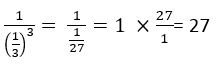• Question 7

So far we have found negative powers of fractions that have a numerator of 1.

We follow the same steps to calculate the negative power of a fraction, that has another number as the numerator.

e.g. Evaluate:This means find the value of:As before, we use our fractions skills to work out:Informally, we can see that finding the reciprocal of the fractions has inverted the original fraction (or turned it upside down!)We can use this 'shortcut' when we need to work out fractions with other negative powers.

e.g. Evaluate:=Use this process to evaluate:Hint: Write this to start:25 /4
EDDIE SAYS
The correct solution is:• Question 8

Which of the options are equivalent to the fraction index below?EDDIE SAYS
So the third option is an intermediate step in our working and the second is the correct, final answer. Well done if you worked that out! Our calculations should look like this:• Question 9

Which of the options are equivalent to the fraction index below?EDDIE SAYS
So we can see that the first option is our final answer and the third has the same value as this. Did you spot that? Our calculations should look like this:• Question 10

Imagine that your friend has evaluated the fraction index below:They have written their answer as:Did they get it right?

Incorrect
EDDIE SAYS
Great job on completing this activity! If you are up for a challenge, move on to practise fractional indices more in our Level 3 activity. This answer is incorrect. Let's check why. Here are the steps in calculating this question:---- OR ----

Sign up for a £1 trial so you can track and measure your child's progress on this activity.

### What is EdPlace?

We're your National Curriculum aligned online education content provider helping each child succeed in English, maths and science from year 1 to GCSE. With an EdPlace account you’ll be able to track and measure progress, helping each child achieve their best. We build confidence and attainment by personalising each child’s learning at a level that suits them.

Get started